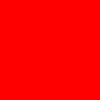Co-founder @ RMOTR

# How to Generate Images With Text Using Python and PIL

Create buttons or banners with a few lines of Python and Pillow.

Last updated: December 7th, 2018

This is a quick guide to show how to draw images with Pillow (recommended PIL's fork). Let's start with a few imports and work the examples step by step:

In :
from PIL import Image, ImageDraw, ImageFont
from utils import display_pillow_image


#### Drawing a simple square image¶

We'll use Image.new to generate a new image of w width and h height, and a specific color; I'll draw a red rectangle (100x100):

In :
w = 100 # 100 pixels wide
h = 100 # 100 pixels high

img = Image.new('RGB', (w, h), color='#FF0000')

In :
display_pillow_image(img)

Out:Color can be specified in hex (#FF0000) or as an RGB array:

In :
img = Image.new('RGB', (350, 70), color=(51, 144, 255))

In :
display_pillow_image(img)

Out:#### Adding text to the image¶

We can create an ImageDraw object to add 2D graphics to the image, for example, text:

In :
img = Image.new('RGB', (350, 70), color=(51, 144, 255))

In :
# create the canvas
canvas = ImageDraw.Draw(img)

In :
canvas.text((10, 10), "Hello World", fill='#FFFFFF')

In :
display_pillow_image(img)

Out:The first tuple passed to text ((10, 10)) is the x and y position of the text, another example:

In :
img = Image.new('RGB', (350, 70), color=(51, 144, 255))

In :
# create the canvas
canvas = ImageDraw.Draw(img)

In :
canvas.text((250, 25), "Hello World", fill='#FFFFFF')

In :
display_pillow_image(img)

Out:#### Customizing the style of text¶

You can use ImageFont to customize the size and the font used. For example, I've included our main typography (Lato) in this project. I can load it locally and use it for the text:

In :
img = Image.new('RGB', (350, 70), color=(51, 144, 255))

In :
# create the canvas
canvas = ImageDraw.Draw(img)

In :
font = ImageFont.truetype('Lato-Bold.ttf', size=24)

In :
canvas.text((10, 10), "Hello World", font=font, fill='#FFFFFF')

In :
display_pillow_image(img)

Out:#### Centering the text¶

There's a useful method of the ImageDraw object that lets you calculate the width and height of the text that you're about to draw. You can use it to compute the correct x and y position of the text.

In :
image_width = 350
image_height = 70

In :
img = Image.new('RGB', (image_width, image_height), color=(51, 144, 255))

In :
# create the canvas
canvas = ImageDraw.Draw(img)

In :
font = ImageFont.truetype('Lato-Bold.ttf', size=24)

In :
text_width, text_height = canvas.textsize('Hello World', font=font)

In :
print(f"Text width: {text_width}")
print(f"Text height: {text_height}")

Text width: 134
Text height: 24

In :
x_pos = int((image_width - text_width) / 2)
y_pos = int((image_height - text_height) / 2)

In :
print(f"X: {x_pos}")
print(f"Y: {y_pos}")

X: 108
Y: 23

In :
canvas.text((x_pos, y_pos), "Hello World", font=font, fill='#FFFFFF')

In :
display_pillow_image(img)

Out:That's it! What other examples can you think about?